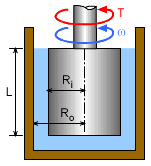Ch 1. Basics Multimedia Engineering Fluids MassDensity IdealGas Law Viscosity SurfaceTension VaporPressure
 Chapter 1. Basics 2. Fluid Statics 3. Kinematics 4. Laws (Integral) 5. Laws (Diff.) 6. Modeling/Similitude 7. Inviscid 8. Viscous 9. External Flow 10. Open-Channel Appendix Basic Math Units Basic Equations Water/Air Tables Sections Search eBooks Dynamics Fluids Math Mechanics Statics Thermodynamics Author(s): Chean Chin Ngo Kurt Gramoll ©Kurt GramollFLUID MECHANICS - CASE STUDY IntroductionRotational Cylindrical Viscometer Students are given a simple device called a rotational cylindrical viscometer, as shown in the figure, and asked to determine the viscosity of an unknown liquid. The outer cylinder is fixed while the inner cylinder is rotating at a constant angular speed of ω by applying a torque T. Questions Derive an equation for the viscosity in terms of angular velocity, ω, torque, T, submerged inner cylinder height, L, inner cylinder radius, Ri, and outer cylinder radius, Ro. Calculate the viscosity value when ω = 55 rev/min, T = 0.9 N-m, L = 0.3 m, Ri = 0.12 m and Ro = 0.13 m. Approach Assume the velocity profile between the container and the rotating cylinder is linear. Neglect the end effects and bottom surface of the cylinder. Assume the liquid is a Newtonian fluid. Assume the force (F) is perpendicular to its moment arm (r), hence the torque is given by T = Fr.

Practice Homework and Test problems now available in the 'Eng Fluids' mobile app
Includes over 250 problems with complete detailed solutions.
Available now at the Google Play Store and Apple App Store.